# Newton's method for quadratic functions

This page discusses how Newton's method fares as a root-finding algorithm for quadratic functions of one variable.

Please beware that this is not the same as using Newton's method for quadratic optimization. Applying Newton's method for optimization of a function of one variable to a quadratic function basically means applying Newton's method as a root-finding algorithm to the derivative of the quadratic function, which is a linear function. And Newton's method should converge in a single step for that function.

Newton's method for quadratic functions is more properly thought of as Newton's method for the optimization of cubic functions.

## Summary

We subdivide into three cases and describe the qualitative behavior of convergence in all cases.

Case on nature of quadratic function Relation between initial guess$x_0$ and root of convergence (assume that we do not start at a root) Monotonicity of convergence Order of convergence Numerical rate of convergence for that order
One root with multiplicity two, i.e., the polynomial is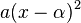$a(x - \alpha)^2$ for some$a,\alpha \in \R$,$a \ne 0$ Converges to the root, but does not reach it in finitely many steps monotonic from the outset 1 1/2
Two distinct real roots, i.e., the polynomial is$a(x - \alpha)(x - \beta)$ for some$a,\alpha,\beta \in \R$,$a \ne 0$,$\alpha < \beta$ Converges to the root it is closer to, but does not reach it in finitely many steps. In particular, converges to$\alpha$ if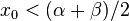$x_0 < (\alpha + \beta)/2$, converges to$\alpha$. If$x_0 > (\alpha + \beta)/2$, converges to$\beta$. monotonic after at most one initial step 2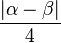$\frac{|\alpha - \beta|}{4}$
No real root No root to converge to, chaotic behavior -- -- --

## One root with multiplicity two

### Baby case

We consider the prototype function:$f(x) := x^2$

The iteration here gives:$x_{+1} = \frac{x_k}{2}$

In other words, each guess is half of the preceding guess. We therefore find that the guesses have linear convergence to the true root, 0, with a convergence rate of 1/2.

### General case

The general case can be dealt with algebraically in a manner similar to the baby case: we note that affine transformations on the domain prior to applying the function, and scaling after applying the function, do not affect the qualitative behavior of Newton's method.

Alternatively, we can deal with the general case separately.

## Two distinct real roots

### Baby case

We consider the prototype function:$f(x) := x^2 - 1$

The iteration here gives: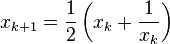$x_{k+1}= \frac{1}{2}\left(x_k+ \frac{1}{x_k}\right)$

We have the following cases (we ignore the cases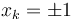$x_k = \pm 1$ because if that happened, the algorithm would terminate):

Case for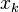$x_k$ Conclusion for$x_n$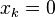$x_k = 0$ The algorithm encounters failure.$0 < x_k < 1$$x_{k+1} > 1$. In other words, applying Newton's method causes us to overcorrect in our move towards the root and land up on the other side of the root. This can be seen both algebraically and geometrically.$x_k > 1$$1 < x_{k+1} < x_k$. In other words, applying Newton's method moves us towards the root, but not all the way, i.e., we don't move enough. This is due to the nature of the curvature of the quadratic function, and can be seen both geometrically and algebraically.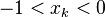$-1 < x_k < 0$$x_{k+1} < -1$. In other words, applying Newton's method causes us to overcorrect in our move towards the root and land up on the other side of the root.$x_{n-1} < -1$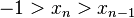$-1 > x_n > x_{n-1}$. In other words, applying Newton's method moves us towards the root, but not all the way, i.e., we don't move enough. This is due to the nature of the curvature of the quadratic function, and can be seen both geometrically and algebraically.

We are now in a position to piece together the long-run convergence behavior based on starting point (we exclude the cases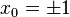$x_0 = \pm 1$, where the function is already at the point where it should be).

Case for$x_0$ Root that the algorithm converges to Explanation$x_0 = 0$ The algorithm encounters failure.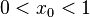$0 < x_0 < 1$ 1$x_1 > 1$, and the sequence then monotonically decreases, converging to 1 (reason same as for the next case).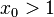$x_0 > 1$ 1 The sequence is monotonically decreasing but bounded from below by 1 (because of how the iteration works at each step, as described in the preceding table). Since any monotonically decreasing sequence bounded from below must converge, the sequence must converge. Further, the limit must be a fixed point of the iteration, and also must be at least 1. This forces the limit to be 1.$-1 < x_0 < 0$ -1$x_1 < 1$, and the sequence then monotonically increases, converging to -1 (reason same as for the next case).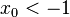$x_0 < -1$ -1 The sequence is monotonically increasing but bounded from above by -1 (because of how the iteration works at each step, as described in the preceding table). Since any monotonically increasing sequence bounded from above must converge, the sequence must converge. Further, the limit must be a fixed point of the iteration, and also must be at most -1. This forces the limit to be -1.

We notice a few nice aspects of this case:

• Either we fail right at the outset, or we converge to a root.
• In all cases, the root we converge to is the root closer to the original starting point.
• The convergence to the root is eventually monotonic. In fact, it is either monotonic from the outset or monotonic from one step after the outset.

These aspects fail for more complicated functions, including polynomials of higher degree.

It also turns out that the order of convergence is 2, with the rate of convergence 1/2 (we get the same order and rate of convergence to both roots, and independent of starting point). In other words, convergence to the root is quadratic. We make two cases:

• Convergence to the root 1: We have: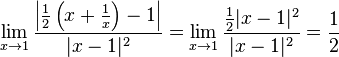$\lim_{x \to 1} \frac{\left|\frac{1}{2}\left(x + \frac{1}{x}\right) - 1\right|}{|x - 1|^2} = \lim_{x \to 1} \frac{\frac{1}{2}|x - 1|^2}{|x - 1|^2} = \frac{1}{2}$

• Convergence to the root -1: We have:$\lim_{x \to -1} \frac{\left|\frac{1}{2}\left(x + \frac{1}{x}\right) - (-1)\right|}{|x - (-1)|^2} = \lim_{x \to 1} \frac{\frac{1}{2}|x + 1|^2}{|x + 1|^2} = \frac{1}{2}$

### General case

We can reduce the general case to the baby case by applying an affine transformation to the domain and a scaling to the function. These do not affect the qualitative behavior of Newton's method.

Alternatively, we could carry out similar calculations to those above for the general case.

## No real root

### Baby case

Consider the prototype function:$f(x) := x^2 + 1$

The iteration here gives:$x_{k+1}= \frac{1}{2}\left(x_k - \frac{1}{x_k}\right)$

The dynamics in this case are complicated and chaotic. Roughly speaking, we have the following cases.

Starting point What happens after enough iterations to transition to another case
We get to zero. The algorithm fails and terminates. Note that because each output can come from at most two inputs in each stage of the iteration, only countably many inputs will get to exactly zero. Therefore, most inputs will not reach this stage.
We are at a positive number$x$ greater than 1 After enough iterations, we get to a number at least equal to 0, but less than 1. If we reach zero, then the algorithm fails. If we reach a number strictly between 0 and 1, then we get to the next case.
We are at a positive number$x$ in the interval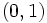$(0,1)$. In the next iteration, we get to a negative number. This could either be a number between -1 and 0, or a number less than -1. Note that if we get to -1, then the next step gives 0, and causes the algorithm to terminate. So the interesting cases are that we get to a number between -1 and 0, and we get to a number less than -1.
We are at a negative number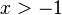$x > -1$. In the next iteration, we get to a positive number. This could be either between 0 and 1 or greater than 1. If we get to exactly 1, the algorithm fails in the next iteration when we get to 0. The interesting cases are therefore the interval$(0,1)$ and numbers greater than 1.
We are at a negative number$x < -1$. After enough iterations, we get to a number at most equal to 0, but greater than 1. If we reach zero, then the algorithm fails. If we reach a number strictly between -1 and 0, then we get to the previous case.

### General case

We can reduce the general case to the baby case by applying an affine transformation to the domain and a scaling to the function. These do not affect the qualitative behavior of Newton's method.

Alternatively, we could carry out similar calculations to those above for the general case.